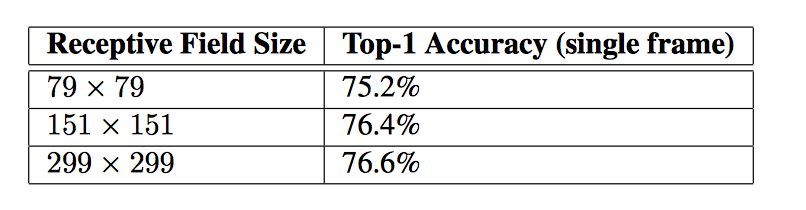• 时间:

• 为什么提出
• 提出的背景
• 基本思想及其过程
• 通用设计原则
• 利用扩大滤波器尺寸来分解卷积
• 辅助分类器的实用性
• 网格尺寸减少很有效
• InceptionV3
• 标签平滑的模型正则化
• 训练方法
• 低分辨率输入上的表现

为什么提出？

提出的背景

• 由于之前都关注的是网络的准确率，但是要真正运用深度学习，更应该关注如何能运用他，也就是要效率高，快。

基本思想及其过程

通用设计原则

1.避免代表性的瓶颈，特别是网络的早期。应该避免极端压缩的瓶颈，通常表示大小应该从输入到输出平缓的减小。
2.更高维表达更容易在网络中局部处理。增加卷积网络中的激活数量有利于加速训练。
3.在没有太多表达能力的损失情况下，空间聚合可以在更低维的嵌入中进行。比如进行3x3卷积时，其可以在空间聚合没有预期严重的不利影响之前减少输入表达的维数。

4.平衡网络的宽度和深度。也就是平衡每个阶段的滤波器的数量以及网络的深度。增加滤波器的数量和网络的深度有利于得到一个高质量的网络，因为他们分散了计算的预算。

利用扩大滤波器尺寸来分解卷积

（1）分解到更小的卷积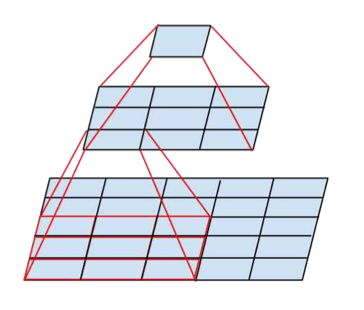（2）空间分解成不对称卷积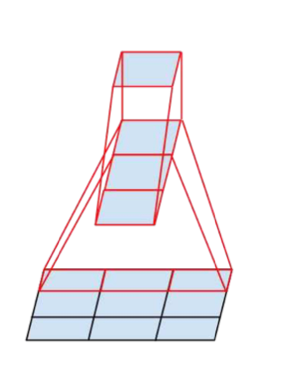辅助分类器的实用性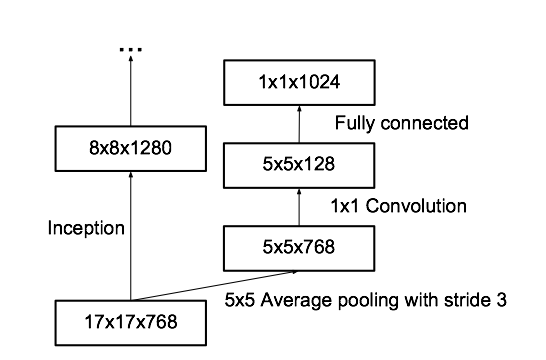网格尺寸减少很有效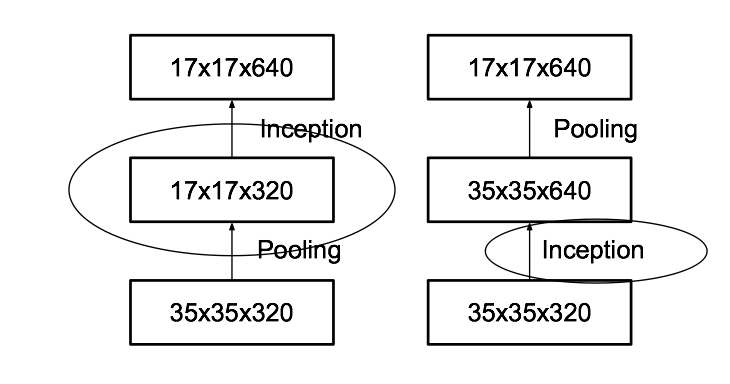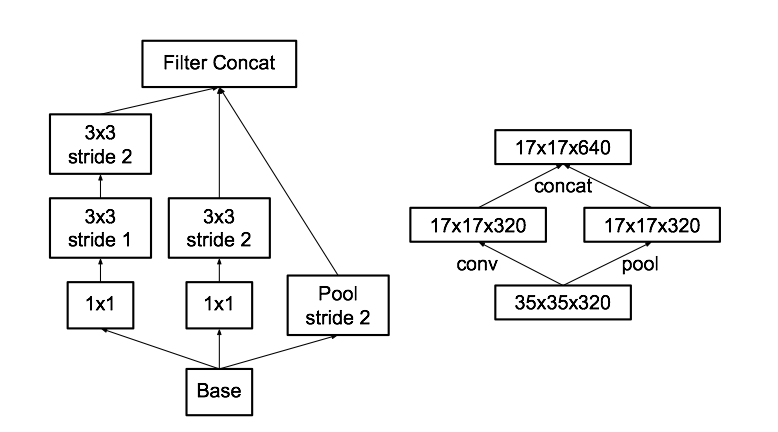InceptionV3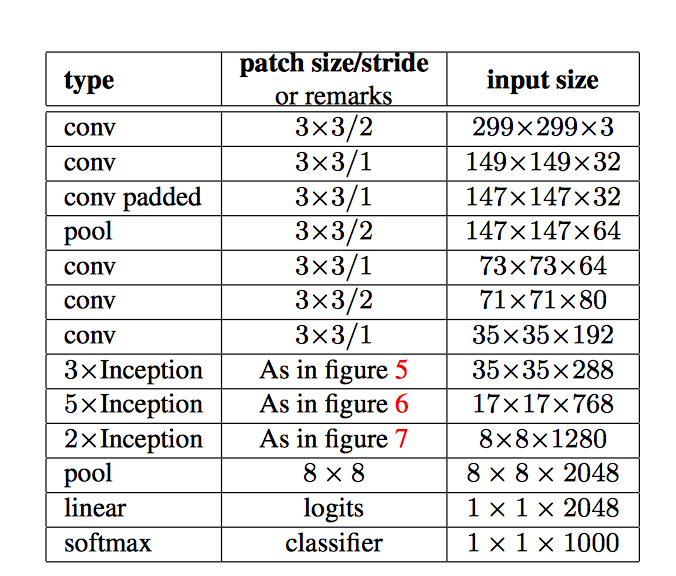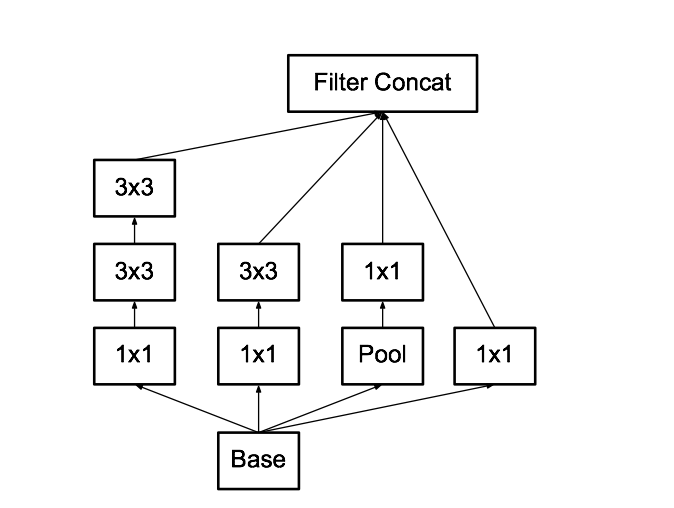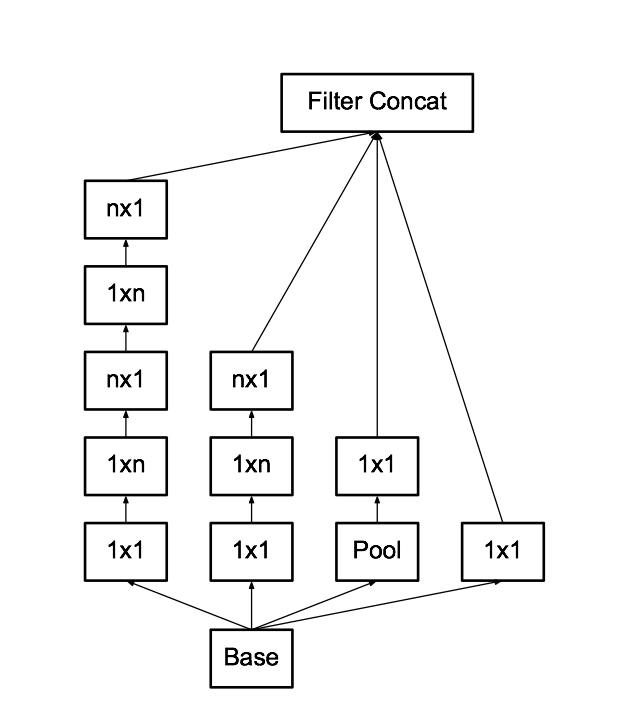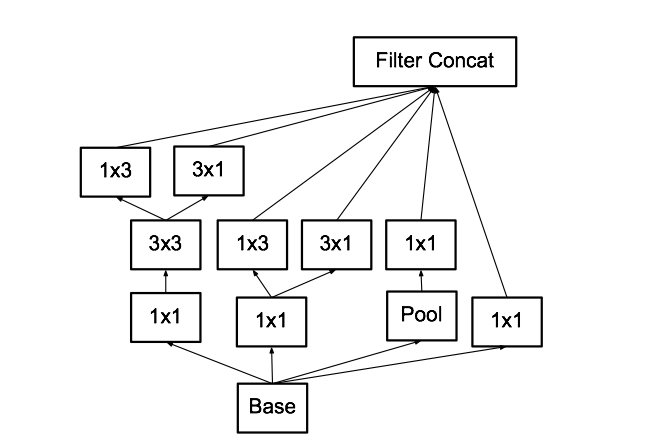标签平滑的模型正则化

q(k)=(1ϵ)δk,y+ϵu(k)$q'(k)=(1-\epsilon)\delta_{k,y}+\epsilon{u(k)}$

q(k)=(1ϵ)δk,y+ϵK$q'(k)=(1-\epsilon)\delta_{k,y}+\frac{\epsilon}{K}$

H(q,p)=Kk=1q(k)logp(k)=(1ϵ)H(q,p)+ϵH(u,p)$H(q',p)=-\sum^{K}_{k=1}q'(k)logp(k)=(1-\epsilon)H(q,p)+\epsilon{H(u,p)}$

低分辨率输入上的表现

1. 299x299感受野，利用步长为2且在第一层后就使用最大池化
2. 151x151感受野，利用步长为1且在第一层厚就使用最大池化
3. 79x79感受野，利用步长为1且在第一层之后不使用池化操作